# R And The Search For Another Earth

## R And The Search For Another Earth

Updated: Jul 15, 2015

## R And The Search For Another Earth

Intro

For this project, my goal was to analyze the NASA Exoplanet Archive dataset in order to predict the likelihood that a Kepler Object of Interest (KOI, potential planet) is indeed a planet.

Background

Before getting into the detail of my analysis, some background information will be helpful. The data I used for this project comes from the NASA Exoplanet Archive. NASA does a good job at describing the archive. The following is from the archive's "About" page, http://exoplanetarchive.ipac.caltech.edu/docs/intro.html :

The NASA Exoplanet Archive is an online astronomical exoplanet and stellar catalog and data service that collates and cross-correlates astronomical data and information on exoplanets and their host stars and provides tools to work with these data. The Exoplanet Archive is dedicated to collecting and serving important public data sets involved in the search for and characterization of extrasolar planets and their host stars. The data include stellar parameters (such as positions, magnitudes, and temperatures), exoplanet parameters (such as masses and orbital parameters) and discovery/characterization data (such as published radial velocity curves, photometric light curves, images, and spectra).

Basically, the website host a large dataset that contains all kinds of information about KOIs. This information ranges from easy-to-interpret variables, such as planetary mass and radius, to obscure and hard-to-interpret variables such as fit parameters from various astrophysical models. Here is a link to the page explaining what all the different variables mean: http://exoplanetarchive.ipac.caltech.edu/docs/API_kepcandidate_columns.html. These variables are obtained through multiple experiments and models and formed into an aggregate set. The most important variable in the dataset is the KOI Disposition, which has values of "Candidate", "Confirmed", and "False Positive". These values indicate the Kepler Missions determination of whether a KOI is indeed a planet or a false positive. The "Candidate" value indicates that a KOI has not been confirmed as a planet or a false positive. So, this dataset seems perfect for training a logisitic regression model using the data points with a KOI Disposition of "Confirmed" and "False Positive" as the training set. Then, once the model is built, the KOI Disposition of data points with a KOI Disposition of "Candidate" can be predicted to be either "Confirmed" or "False Positive". This analysis could be used to select "Candidate" KOIs for confirmation that have a high probability of being "Confirmed". This is beneficial because in order to confirm these KOIs as planets, satelite or ground-based telescopes must use up their finite and expensive resources to double check these KOIs. By double checking the KOIs with the highest probability of being "Confirmed" NASA can cut down on the effective cost per planet confirmation/discovery.

Analysis

To begin my analysis, I first had to download the KOI Cumulative List data set, which can be found here: http://exoplanetarchive.ipac.caltech.edu/cgi-bin/TblView/nph-tblView?app=ExoTbls&config=cumulative. I cheated a little and opened the .csv file in excel and cleaned it up some before importing to R. Once imported into R, I looked at the summary.

``````## randomForest 4.6-10
## Type rfNews() to see new features/changes/bug fixes.
##
## Attaching package: 'zoo'
##
## The following objects are masked from 'package:base':
##
##     as.Date, as.Date.numeric
##
## Type 'citation("pROC")' for a citation.
##
## Attaching package: 'pROC'
##
## The following objects are masked from 'package:stats':
##
##     cov, smooth, var``````
``````#import data

#summary of data
summary(kepler)``````
``````##        koi_disposition   koi_period          koi_depth
##  CANDIDATE     :3185   Min.   :     0.24   Min.   :     5.9
##  CONFIRMED     : 993   1st Qu.:     3.56   1st Qu.:   195.8
##  FALSE POSITIVE:3170   Median :    11.26   Median :   471.9
##                        Mean   :    78.12   Mean   : 15301.5
##                        3rd Qu.:    43.15   3rd Qu.:  1301.7
##                        Max.   :129995.78   Max.   :961488.0
##                                            NA's   :284
##   koi_duration      koi_impact          koi_incl        koi_sma
##  Min.   : 0.250   Min.   :  0.0000   Min.   : 6.88   Min.   : 0.00580
##  1st Qu.: 2.399   1st Qu.:  0.1505   1st Qu.:85.94   1st Qu.: 0.04400
##  Median : 3.645   Median :  0.4073   Median :88.81   Median : 0.09205
##  Mean   : 5.130   Mean   :  1.1874   Mean   :83.43   Mean   : 0.22077
##  3rd Qu.: 5.975   3rd Qu.:  0.8220   3rd Qu.:89.95   3rd Qu.: 0.21862
##  Max.   :93.160   Max.   :100.9710   Max.   :89.95   Max.   :44.98920
##                   NA's   :284        NA's   :284     NA's   :284
##  Min.   :    0.91   Min.   : 0.00228   Min.   :     0.14
##  1st Qu.:    7.26   1st Qu.: 0.01330   1st Qu.:     1.42
##  Median :   19.07   Median : 0.02164   Median :     2.29
##  Mean   :   85.86   Mean   : 0.77384   Mean   :   132.07
##  3rd Qu.:   51.56   3rd Qu.: 0.05537   3rd Qu.:     7.91
##  Max.   :79614.00   Max.   :99.98200   Max.   :109061.00
##  NA's   :284        NA's   :284        NA's   :284
##  Min.   :   25.0   Min.   : 2661   Min.   :0.146   Min.   :  0.116
##  1st Qu.:  522.8   1st Qu.: 5324   1st Qu.:4.332   1st Qu.:  0.816
##  Median :  820.5   Median : 5787   Median :4.466   Median :  0.963
##  Mean   :  986.0   Mean   : 5714   Mean   :4.370   Mean   :  1.445
##  3rd Qu.: 1241.2   3rd Qu.: 6131   3rd Qu.:4.554   3rd Qu.:  1.164
##  Max.   :14225.0   Max.   :11086   Max.   :5.283   Max.   :149.058
##  NA's   :284       NA's   :284     NA's   :284     NA's   :284
##    koi_smass            ra             dec          koi_kepmag
##  Min.   :0.0940   Min.   :279.9   Min.   :36.58   Min.   : 6.966
##  1st Qu.:0.8330   1st Qu.:288.5   1st Qu.:40.77   1st Qu.:13.530
##  Median :0.9690   Median :292.2   Median :43.72   Median :14.601
##  Mean   :0.9902   Mean   :292.0   Mean   :43.83   Mean   :14.328
##  3rd Qu.:1.0770   3rd Qu.:295.9   3rd Qu.:46.73   3rd Qu.:15.344
##  Max.   :3.6440   Max.   :301.7   Max.   :52.34   Max.   :20.003
##  NA's   :284
##     koi_gmag         koi_rmag         koi_imag         koi_zmag
##  Min.   : 7.225   Min.   : 7.101   Min.   : 7.641   Min.   : 7.657
##  1st Qu.:14.014   1st Qu.:13.479   1st Qu.:13.371   1st Qu.:13.346
##  Median :15.143   Median :14.553   Median :14.373   Median :14.302
##  Mean   :14.903   Mean   :14.284   Mean   :14.132   Mean   :14.047
##  3rd Qu.:15.971   3rd Qu.:15.298   3rd Qu.:15.084   3rd Qu.:14.960
##  Max.   :21.150   Max.   :19.960   Max.   :19.900   Max.   :17.403
##  NA's   :32       NA's   :8        NA's   :107      NA's   :460
##     koi_jmag         koi_hmag         koi_kmag        koi_count
##  Min.   : 5.393   Min.   : 4.854   Min.   : 4.718   Min.   :1.000
##  1st Qu.:12.330   1st Qu.:11.988   1st Qu.:11.919   1st Qu.:1.000
##  Median :13.293   Median :12.892   Median :12.806   Median :1.000
##  Mean   :13.050   Mean   :12.673   Mean   :12.596   Mean   :1.461
##  3rd Qu.:13.994   3rd Qu.:13.579   3rd Qu.:13.509   3rd Qu.:2.000
##  Max.   :17.372   Max.   :17.615   Max.   :17.038   Max.   :7.000
##  NA's   :21       NA's   :21       NA's   :21
##    koi_insol          koi_srho        koi_model_snr      koi_fpflag_nt
##  Min.   :      0   Min.   :  0.0001   Min.   :    1.00   Min.   :0.0000
##  1st Qu.:     18   1st Qu.:  0.3526   1st Qu.:   14.30   1st Qu.:0.0000
##  Median :    107   Median :  1.3860   Median :   27.45   Median :0.0000
##  Mean   :   5396   Mean   : 12.8930   Mean   :  215.16   Mean   :0.1179
##  3rd Qu.:    562   3rd Qu.:  3.8115   3rd Qu.:   78.30   3rd Qu.:0.0000
##  Max.   :9678287   Max.   :989.5966   Max.   :12808.40   Max.   :1.0000
##  NA's   :284       NA's   :284        NA's   :284
##  koi_fpflag_ss    koi_fpflag_co     koi_fpflag_ec    koi_ldm_coeff1
##  Min.   :0.0000   Min.   :0.00000   Min.   :0.0000   Min.   :0.1161
##  1st Qu.:0.0000   1st Qu.:0.00000   1st Qu.:0.0000   1st Qu.:0.3250
##  Median :0.0000   Median :0.00000   Median :0.0000   Median :0.3717
##  Mean   :0.1116   Mean   :0.08628   Mean   :0.1076   Mean   :0.3964
##  3rd Qu.:0.0000   3rd Qu.:0.00000   3rd Qu.:0.0000   3rd Qu.:0.4488
##  Max.   :1.0000   Max.   :1.00000   Max.   :1.0000   Max.   :0.9122
##                                                      NA's   :284
##  koi_ldm_coeff2
##  Min.   :-0.1135
##  1st Qu.: 0.2375
##  Median : 0.2814
##  Mean   : 0.2617
##  3rd Qu.: 0.3005
##  Max.   : 0.4467
##  NA's   :284``````

After a quick glance at the summary, I removed some outliers and found some near zero variance variables.

``````#clean data up
kepler\$koi_disposition = as.character(kepler\$koi_disposition)
kepler = na.omit(kepler)

#remove near zero variance variables
idx = 1
tempList = numeric(0)
for (i in 2:length(kepler[1,])){

if (var(kepler[,i]) < .5){

tempList[idx] = i
idx = idx + 1

}
}``````

Then, I remove the near zero variance variables and create various data partitions for the modeling and graphing. I split the data into two main sets. "trainingSet" contains all the "Confirmed" and "False Positive" data points, and the "newSet" set contains the unconfirmed "Candidate" data points. Furthermore, I randomly split the training set into train, validation, and test sets. Finally, I made a centered and scaled copy of all the different data splits and converted the response variable to 0 or 1.

``````#create a centered and scaled dataset
keplerCS = kepler[,-tempList]
kepler = kepler[,-tempList]

keplerCS = data.frame(scale(keplerCS[,-1], center = TRUE, scale = TRUE))
keplerCS = cbind(koi_disposition = kepler\$koi_disposition, keplerCS)
keplerCS\$koi_disposition = as.character(keplerCS\$koi_disposition)

#separate out training validation test and new sets
newSet = kepler[kepler\$koi_disposition == "CANDIDATE",]
trainingSet = kepler[kepler\$koi_disposition != "CANDIDATE",]
newSetCS = keplerCS[keplerCS\$koi_disposition == "CANDIDATE",]
trainingSetCS = keplerCS[keplerCS\$koi_disposition != "CANDIDATE",]

indices = sample(1:length(trainingSet[,1]), size=length(trainingSet[,1]),
replace = FALSE, prob = NULL)

fullSet = trainingSet
fullSetCS = trainingSetCS

testSet = fullSet[indices[1:700],]
finalSet =  fullSet[indices[701:1400],]
trainingSet = fullSet[-indices[1:1400],]

testSetCS = fullSetCS[indices[1:700],]
finalSetCS =  fullSetCS[indices[701:1400],]
trainingSetCS = fullSetCS[-indices[1:1400],]

test_koi_disp = ifelse(testSet\$koi_disposition == "CONFIRMED", 1, 0)
testSet\$koi_disposition = test_koi_disp

train_koi_disp = ifelse(trainingSet\$koi_disposition == "CONFIRMED", 1, 0)
trainingSet\$koi_disposition = train_koi_disp

full_koi_disp = ifelse(fullSet\$koi_disposition == "CONFIRMED", 1, 0)
fullSet\$koi_disposition = full_koi_disp

test_koi_dispCS = ifelse(testSetCS\$koi_disposition == "CONFIRMED", 1, 0)
testSetCS\$koi_disposition = test_koi_dispCS

train_koi_dispCS = ifelse(trainingSetCS\$koi_disposition == "CONFIRMED", 1,0)
trainingSetCS\$koi_disposition = train_koi_dispCS

full_koi_dispCS = ifelse(fullSetCS\$koi_disposition == "CONFIRMED", 1, 0)
fullSetCS\$koi_disposition = full_koi_dispCS``````

Next, I need to find an optimal subset of predictor variables to feed to the model.

``````#find best subset of predictors
subs <- regsubsets(koi_disposition ~ .,nvmax = length(names(trainingSetCS)), data = trainingSetCS)
summary(subs)``````
``````## Subset selection object
## Call: regsubsets.formula(koi_disposition ~ ., nvmax = length(names(trainingSetCS)),
##     data = trainingSetCS)
## 25 Variables  (and intercept)
##               Forced in Forced out
## koi_period        FALSE      FALSE
## koi_depth         FALSE      FALSE
## koi_duration      FALSE      FALSE
## koi_impact        FALSE      FALSE
## koi_incl          FALSE      FALSE
## koi_dor           FALSE      FALSE
## koi_ror           FALSE      FALSE
## koi_teq           FALSE      FALSE
## koi_steff         FALSE      FALSE
## ra                FALSE      FALSE
## dec               FALSE      FALSE
## koi_kepmag        FALSE      FALSE
## koi_gmag          FALSE      FALSE
## koi_rmag          FALSE      FALSE
## koi_imag          FALSE      FALSE
## koi_zmag          FALSE      FALSE
## koi_jmag          FALSE      FALSE
## koi_hmag          FALSE      FALSE
## koi_kmag          FALSE      FALSE
## koi_count         FALSE      FALSE
## koi_insol         FALSE      FALSE
## koi_srho          FALSE      FALSE
## koi_model_snr     FALSE      FALSE
## 1 subsets of each size up to 25
## Selection Algorithm: exhaustive
##           koi_period koi_depth koi_duration koi_impact koi_incl koi_dor
## 1  ( 1 )  " "        " "       " "          " "        " "      " "
## 2  ( 1 )  " "        " "       " "          " "        "*"      " "
## 3  ( 1 )  "*"        " "       " "          " "        "*"      " "
## 4  ( 1 )  "*"        " "       " "          " "        "*"      " "
## 5  ( 1 )  "*"        "*"       " "          " "        "*"      " "
## 6  ( 1 )  "*"        " "       "*"          " "        "*"      " "
## 7  ( 1 )  "*"        " "       "*"          " "        "*"      " "
## 8  ( 1 )  "*"        "*"       "*"          "*"        " "      " "
## 9  ( 1 )  "*"        "*"       "*"          "*"        " "      " "
## 10  ( 1 ) "*"        "*"       "*"          "*"        " "      "*"
## 11  ( 1 ) "*"        "*"       "*"          "*"        " "      "*"
## 12  ( 1 ) "*"        "*"       "*"          "*"        " "      "*"
## 13  ( 1 ) "*"        "*"       "*"          "*"        " "      "*"
## 14  ( 1 ) "*"        "*"       "*"          "*"        " "      "*"
## 15  ( 1 ) "*"        "*"       "*"          "*"        " "      "*"
## 16  ( 1 ) "*"        "*"       "*"          "*"        " "      "*"
## 17  ( 1 ) "*"        "*"       "*"          "*"        " "      "*"
## 18  ( 1 ) "*"        "*"       "*"          "*"        " "      "*"
## 19  ( 1 ) "*"        "*"       "*"          "*"        " "      "*"
## 20  ( 1 ) "*"        "*"       "*"          "*"        " "      "*"
## 21  ( 1 ) "*"        "*"       "*"          "*"        " "      "*"
## 22  ( 1 ) "*"        "*"       "*"          "*"        " "      "*"
## 23  ( 1 ) "*"        "*"       "*"          "*"        "*"      "*"
## 24  ( 1 ) "*"        "*"       "*"          "*"        "*"      "*"
## 25  ( 1 ) "*"        "*"       "*"          "*"        "*"      "*"
## 1  ( 1 )  " "     " "      " "     " "       " "      " " " " " "
## 2  ( 1 )  " "     " "      " "     " "       " "      " " " " " "
## 3  ( 1 )  " "     " "      " "     " "       " "      " " " " " "
## 4  ( 1 )  " "     " "      "*"     " "       " "      " " " " " "
## 5  ( 1 )  " "     " "      "*"     " "       " "      " " " " " "
## 6  ( 1 )  " "     " "      "*"     " "       " "      " " " " " "
## 7  ( 1 )  " "     " "      "*"     " "       " "      " " " " " "
## 8  ( 1 )  "*"     " "      "*"     " "       " "      " " " " " "
## 9  ( 1 )  "*"     " "      "*"     " "       " "      " " " " " "
## 10  ( 1 ) "*"     " "      "*"     " "       " "      " " " " " "
## 11  ( 1 ) "*"     " "      "*"     " "       " "      " " "*" " "
## 12  ( 1 ) "*"     " "      "*"     " "       " "      "*" "*" " "
## 13  ( 1 ) "*"     " "      "*"     "*"       " "      " " "*" " "
## 14  ( 1 ) "*"     " "      "*"     "*"       "*"      " " "*" "*"
## 15  ( 1 ) "*"     " "      "*"     "*"       "*"      "*" "*" " "
## 16  ( 1 ) "*"     " "      "*"     "*"       "*"      "*" "*" " "
## 17  ( 1 ) "*"     " "      "*"     "*"       "*"      "*" "*" " "
## 18  ( 1 ) "*"     " "      "*"     "*"       "*"      "*" "*" "*"
## 19  ( 1 ) "*"     " "      "*"     "*"       "*"      "*" "*" " "
## 20  ( 1 ) "*"     " "      "*"     "*"       "*"      "*" "*" "*"
## 21  ( 1 ) "*"     " "      "*"     "*"       "*"      "*" "*" "*"
## 22  ( 1 ) "*"     "*"      "*"     "*"       "*"      "*" "*" "*"
## 23  ( 1 ) "*"     "*"      "*"     "*"       "*"      "*" "*" "*"
## 24  ( 1 ) "*"     "*"      "*"     "*"       "*"      "*" "*" "*"
## 25  ( 1 ) "*"     "*"      "*"     "*"       "*"      "*" "*" "*"
##           koi_gmag koi_rmag koi_imag koi_zmag koi_jmag koi_hmag koi_kmag
## 1  ( 1 )  " "      " "      " "      " "      " "      " "      " "
## 2  ( 1 )  " "      " "      " "      " "      " "      " "      " "
## 3  ( 1 )  " "      " "      " "      " "      " "      " "      " "
## 4  ( 1 )  " "      " "      " "      " "      " "      " "      " "
## 5  ( 1 )  " "      " "      " "      " "      " "      " "      " "
## 6  ( 1 )  " "      " "      "*"      " "      " "      " "      " "
## 7  ( 1 )  " "      "*"      " "      " "      " "      " "      " "
## 8  ( 1 )  " "      "*"      " "      " "      " "      " "      " "
## 9  ( 1 )  " "      "*"      " "      " "      " "      " "      " "
## 10  ( 1 ) " "      "*"      " "      " "      " "      " "      " "
## 11  ( 1 ) " "      "*"      " "      " "      " "      " "      " "
## 12  ( 1 ) " "      "*"      " "      " "      " "      " "      " "
## 13  ( 1 ) " "      "*"      " "      " "      "*"      " "      " "
## 14  ( 1 ) " "      " "      " "      " "      "*"      " "      " "
## 15  ( 1 ) " "      "*"      " "      " "      " "      "*"      " "
## 16  ( 1 ) "*"      "*"      " "      " "      " "      "*"      " "
## 17  ( 1 ) "*"      "*"      " "      " "      " "      "*"      " "
## 18  ( 1 ) "*"      "*"      " "      " "      " "      "*"      " "
## 19  ( 1 ) "*"      "*"      " "      "*"      "*"      "*"      " "
## 20  ( 1 ) "*"      "*"      "*"      "*"      " "      "*"      " "
## 21  ( 1 ) "*"      "*"      "*"      "*"      "*"      "*"      " "
## 22  ( 1 ) "*"      "*"      "*"      "*"      "*"      "*"      " "
## 23  ( 1 ) "*"      "*"      "*"      "*"      "*"      "*"      " "
## 24  ( 1 ) "*"      "*"      "*"      "*"      "*"      "*"      " "
## 25  ( 1 ) "*"      "*"      "*"      "*"      "*"      "*"      "*"
##           koi_count koi_insol koi_srho koi_model_snr
## 1  ( 1 )  "*"       " "       " "      " "
## 2  ( 1 )  "*"       " "       " "      " "
## 3  ( 1 )  "*"       " "       " "      " "
## 4  ( 1 )  "*"       " "       " "      " "
## 5  ( 1 )  "*"       " "       " "      " "
## 6  ( 1 )  "*"       " "       " "      " "
## 7  ( 1 )  "*"       "*"       " "      " "
## 8  ( 1 )  "*"       " "       " "      " "
## 9  ( 1 )  "*"       "*"       " "      " "
## 10  ( 1 ) "*"       "*"       " "      " "
## 11  ( 1 ) "*"       "*"       " "      " "
## 12  ( 1 ) "*"       "*"       " "      " "
## 13  ( 1 ) "*"       "*"       " "      " "
## 14  ( 1 ) "*"       "*"       " "      " "
## 15  ( 1 ) "*"       "*"       " "      " "
## 16  ( 1 ) "*"       "*"       " "      " "
## 17  ( 1 ) "*"       "*"       " "      "*"
## 18  ( 1 ) "*"       "*"       " "      "*"
## 19  ( 1 ) "*"       "*"       " "      "*"
## 20  ( 1 ) "*"       "*"       " "      "*"
## 21  ( 1 ) "*"       "*"       " "      "*"
## 22  ( 1 ) "*"       "*"       " "      "*"
## 23  ( 1 ) "*"       "*"       " "      "*"
## 24  ( 1 ) "*"       "*"       "*"      "*"
## 25  ( 1 ) "*"       "*"       "*"      "*"``````
``plot(summary(subs)\$adjr2)``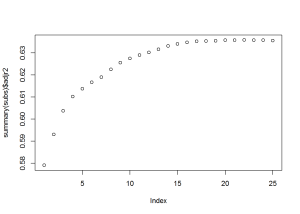``plot(summary(subs)\$cp)``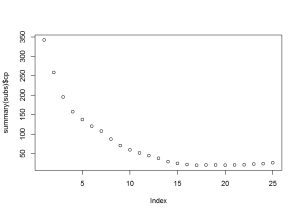``plot(summary(subs)\$bic)``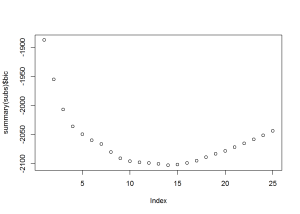``summary(subs)\$which``
``````##    (Intercept) koi_period koi_depth koi_duration koi_impact koi_incl
## 1         TRUE      FALSE     FALSE        FALSE      FALSE    FALSE
## 2         TRUE      FALSE     FALSE        FALSE      FALSE     TRUE
## 3         TRUE       TRUE     FALSE        FALSE      FALSE     TRUE
## 4         TRUE       TRUE     FALSE        FALSE      FALSE     TRUE
## 5         TRUE       TRUE      TRUE        FALSE      FALSE     TRUE
## 6         TRUE       TRUE     FALSE         TRUE      FALSE     TRUE
## 7         TRUE       TRUE     FALSE         TRUE      FALSE     TRUE
## 8         TRUE       TRUE      TRUE         TRUE       TRUE    FALSE
## 9         TRUE       TRUE      TRUE         TRUE       TRUE    FALSE
## 10        TRUE       TRUE      TRUE         TRUE       TRUE    FALSE
## 11        TRUE       TRUE      TRUE         TRUE       TRUE    FALSE
## 12        TRUE       TRUE      TRUE         TRUE       TRUE    FALSE
## 13        TRUE       TRUE      TRUE         TRUE       TRUE    FALSE
## 14        TRUE       TRUE      TRUE         TRUE       TRUE    FALSE
## 15        TRUE       TRUE      TRUE         TRUE       TRUE    FALSE
## 16        TRUE       TRUE      TRUE         TRUE       TRUE    FALSE
## 17        TRUE       TRUE      TRUE         TRUE       TRUE    FALSE
## 18        TRUE       TRUE      TRUE         TRUE       TRUE    FALSE
## 19        TRUE       TRUE      TRUE         TRUE       TRUE    FALSE
## 20        TRUE       TRUE      TRUE         TRUE       TRUE    FALSE
## 21        TRUE       TRUE      TRUE         TRUE       TRUE    FALSE
## 22        TRUE       TRUE      TRUE         TRUE       TRUE    FALSE
## 23        TRUE       TRUE      TRUE         TRUE       TRUE     TRUE
## 24        TRUE       TRUE      TRUE         TRUE       TRUE     TRUE
## 25        TRUE       TRUE      TRUE         TRUE       TRUE     TRUE
## 1    FALSE   FALSE    FALSE   FALSE     FALSE    FALSE FALSE FALSE
## 2    FALSE   FALSE    FALSE   FALSE     FALSE    FALSE FALSE FALSE
## 3    FALSE   FALSE    FALSE   FALSE     FALSE    FALSE FALSE FALSE
## 4    FALSE   FALSE    FALSE    TRUE     FALSE    FALSE FALSE FALSE
## 5    FALSE   FALSE    FALSE    TRUE     FALSE    FALSE FALSE FALSE
## 6    FALSE   FALSE    FALSE    TRUE     FALSE    FALSE FALSE FALSE
## 7    FALSE   FALSE    FALSE    TRUE     FALSE    FALSE FALSE FALSE
## 8    FALSE    TRUE    FALSE    TRUE     FALSE    FALSE FALSE FALSE
## 9    FALSE    TRUE    FALSE    TRUE     FALSE    FALSE FALSE FALSE
## 10    TRUE    TRUE    FALSE    TRUE     FALSE    FALSE FALSE FALSE
## 11    TRUE    TRUE    FALSE    TRUE     FALSE    FALSE FALSE  TRUE
## 12    TRUE    TRUE    FALSE    TRUE     FALSE    FALSE  TRUE  TRUE
## 13    TRUE    TRUE    FALSE    TRUE      TRUE    FALSE FALSE  TRUE
## 14    TRUE    TRUE    FALSE    TRUE      TRUE     TRUE FALSE  TRUE
## 15    TRUE    TRUE    FALSE    TRUE      TRUE     TRUE  TRUE  TRUE
## 16    TRUE    TRUE    FALSE    TRUE      TRUE     TRUE  TRUE  TRUE
## 17    TRUE    TRUE    FALSE    TRUE      TRUE     TRUE  TRUE  TRUE
## 18    TRUE    TRUE    FALSE    TRUE      TRUE     TRUE  TRUE  TRUE
## 19    TRUE    TRUE    FALSE    TRUE      TRUE     TRUE  TRUE  TRUE
## 20    TRUE    TRUE    FALSE    TRUE      TRUE     TRUE  TRUE  TRUE
## 21    TRUE    TRUE    FALSE    TRUE      TRUE     TRUE  TRUE  TRUE
## 22    TRUE    TRUE     TRUE    TRUE      TRUE     TRUE  TRUE  TRUE
## 23    TRUE    TRUE     TRUE    TRUE      TRUE     TRUE  TRUE  TRUE
## 24    TRUE    TRUE     TRUE    TRUE      TRUE     TRUE  TRUE  TRUE
## 25    TRUE    TRUE     TRUE    TRUE      TRUE     TRUE  TRUE  TRUE
##    koi_kepmag koi_gmag koi_rmag koi_imag koi_zmag koi_jmag koi_hmag
## 1       FALSE    FALSE    FALSE    FALSE    FALSE    FALSE    FALSE
## 2       FALSE    FALSE    FALSE    FALSE    FALSE    FALSE    FALSE
## 3       FALSE    FALSE    FALSE    FALSE    FALSE    FALSE    FALSE
## 4       FALSE    FALSE    FALSE    FALSE    FALSE    FALSE    FALSE
## 5       FALSE    FALSE    FALSE    FALSE    FALSE    FALSE    FALSE
## 6       FALSE    FALSE    FALSE     TRUE    FALSE    FALSE    FALSE
## 7       FALSE    FALSE     TRUE    FALSE    FALSE    FALSE    FALSE
## 8       FALSE    FALSE     TRUE    FALSE    FALSE    FALSE    FALSE
## 9       FALSE    FALSE     TRUE    FALSE    FALSE    FALSE    FALSE
## 10      FALSE    FALSE     TRUE    FALSE    FALSE    FALSE    FALSE
## 11      FALSE    FALSE     TRUE    FALSE    FALSE    FALSE    FALSE
## 12      FALSE    FALSE     TRUE    FALSE    FALSE    FALSE    FALSE
## 13      FALSE    FALSE     TRUE    FALSE    FALSE     TRUE    FALSE
## 14       TRUE    FALSE    FALSE    FALSE    FALSE     TRUE    FALSE
## 15      FALSE    FALSE     TRUE    FALSE    FALSE    FALSE     TRUE
## 16      FALSE     TRUE     TRUE    FALSE    FALSE    FALSE     TRUE
## 17      FALSE     TRUE     TRUE    FALSE    FALSE    FALSE     TRUE
## 18       TRUE     TRUE     TRUE    FALSE    FALSE    FALSE     TRUE
## 19      FALSE     TRUE     TRUE    FALSE     TRUE     TRUE     TRUE
## 20       TRUE     TRUE     TRUE     TRUE     TRUE    FALSE     TRUE
## 21       TRUE     TRUE     TRUE     TRUE     TRUE     TRUE     TRUE
## 22       TRUE     TRUE     TRUE     TRUE     TRUE     TRUE     TRUE
## 23       TRUE     TRUE     TRUE     TRUE     TRUE     TRUE     TRUE
## 24       TRUE     TRUE     TRUE     TRUE     TRUE     TRUE     TRUE
## 25       TRUE     TRUE     TRUE     TRUE     TRUE     TRUE     TRUE
##    koi_kmag koi_count koi_insol koi_srho koi_model_snr
## 1     FALSE      TRUE     FALSE    FALSE         FALSE
## 2     FALSE      TRUE     FALSE    FALSE         FALSE
## 3     FALSE      TRUE     FALSE    FALSE         FALSE
## 4     FALSE      TRUE     FALSE    FALSE         FALSE
## 5     FALSE      TRUE     FALSE    FALSE         FALSE
## 6     FALSE      TRUE     FALSE    FALSE         FALSE
## 7     FALSE      TRUE      TRUE    FALSE         FALSE
## 8     FALSE      TRUE     FALSE    FALSE         FALSE
## 9     FALSE      TRUE      TRUE    FALSE         FALSE
## 10    FALSE      TRUE      TRUE    FALSE         FALSE
## 11    FALSE      TRUE      TRUE    FALSE         FALSE
## 12    FALSE      TRUE      TRUE    FALSE         FALSE
## 13    FALSE      TRUE      TRUE    FALSE         FALSE
## 14    FALSE      TRUE      TRUE    FALSE         FALSE
## 15    FALSE      TRUE      TRUE    FALSE         FALSE
## 16    FALSE      TRUE      TRUE    FALSE         FALSE
## 17    FALSE      TRUE      TRUE    FALSE          TRUE
## 18    FALSE      TRUE      TRUE    FALSE          TRUE
## 19    FALSE      TRUE      TRUE    FALSE          TRUE
## 20    FALSE      TRUE      TRUE    FALSE          TRUE
## 21    FALSE      TRUE      TRUE    FALSE          TRUE
## 22    FALSE      TRUE      TRUE    FALSE          TRUE
## 23    FALSE      TRUE      TRUE    FALSE          TRUE
## 24    FALSE      TRUE      TRUE     TRUE          TRUE
## 25     TRUE      TRUE      TRUE     TRUE          TRUE``````

From the plots, it looks like subset 13, 14, or 15, would work best. Using subset 15, I created 3 models: logistic regression, classification tree, and random forest.

``````#test prediction
#MODEL 1: glm
model = glm(koi_disposition ~ . , data=trainingSetCS[,summary(subs)\$which[15,]], family='binomial')``````
``## Warning: glm.fit: fitted probabilities numerically 0 or 1 occurred``
``summary(model)``
``````##
## Call:
## glm(formula = koi_disposition ~ ., family = "binomial", data = trainingSetCS[,
##     summary(subs)\$which[15, ]])
##
## Deviance Residuals:
##     Min       1Q   Median       3Q      Max
## -4.5188  -0.2357  -0.0673   0.0150   3.3765
##
## Coefficients:
##               Estimate Std. Error z value Pr(>|z|)
## (Intercept)   -3.09048    0.25263 -12.233  < 2e-16 ***
## koi_period     8.67353    4.27076   2.031  0.04226 *
## koi_depth     -2.58195    0.87014  -2.967  0.00300 **
## koi_duration  -1.15722    0.21906  -5.283 1.27e-07 ***
## koi_impact   -14.21874    2.13240  -6.668 2.59e-11 ***
## koi_dor      -26.22156    3.65179  -7.180 6.95e-13 ***
## koi_ror       14.15032    2.14695   6.591 4.37e-11 ***
## koi_teq       -2.29169    0.25952  -8.831  < 2e-16 ***
## koi_steff     -1.56063    0.37063  -4.211 2.55e-05 ***
## koi_srad       0.51572    0.10764   4.791 1.66e-06 ***
## ra            -0.23622    0.10211  -2.313  0.02070 *
## dec            0.27351    0.09861   2.774  0.00555 **
## koi_rmag      -4.73568    0.97924  -4.836 1.32e-06 ***
## koi_hmag       4.13194    0.92002   4.491 7.08e-06 ***
## koi_count      2.50930    0.14602  17.184  < 2e-16 ***
## koi_insol      0.51120    0.65852   0.776  0.43758
## ---
## Signif. codes:  0 '***' 0.001 '**' 0.01 '*' 0.05 '.' 0.1 ' ' 1
##
## (Dispersion parameter for binomial family taken to be 1)
##
##     Null deviance: 2551.06  on 2197  degrees of freedom
## Residual deviance:  708.91  on 2182  degrees of freedom
## AIC: 740.91
##
## Number of Fisher Scoring iterations: 9``````
``````#test prediction
preda = predict(model,
newdata=testSetCS,
type='response')

pred1 = ifelse(preda > 0.9, 1, 0)

table(testSetCS\$koi_disposition, pred1)``````
``````##    pred1
##       0   1
##   0 505   3
##   1  71 121``````
``sum(diag(table(pred1, testSetCS\$koi_disposition))) / sum(table(pred1, testSetCS\$koi_disposition))``
``##  0.8942857``
``````roc(testSetCS\$koi_disposition, pred1, main="Statistical comparison",
percent=TRUE, col="#1c61b6")``````
``````##
## Call:
## roc.default(response = testSetCS\$koi_disposition, predictor = pred1,     percent = TRUE, main = "Statistical comparison", col = "#1c61b6")
##
## Data: pred1 in 508 controls (testSetCS\$koi_disposition 0) < 192 cases (testSetCS\$koi_disposition 1).
## Area under the curve: 81.22%``````
``````#Model 2: random forest

fit.rf = randomForest(koi_disposition ~ . , data=trainingSetCS[,summary(subs)\$which[15,]])``````
``````## Warning in randomForest.default(m, y, ...): The response has five or fewer
## unique values.  Are you sure you want to do regression?``````
``````predb = predict(fit.rf,
newdata=testSetCS,
type='response')

pred2 = ifelse(predb > 0.9, 1, 0)

table(testSetCS\$koi_disposition, pred2)``````
``````##    pred2
##       0   1
##   0 504   4
##   1  64 128``````
``sum(diag(table(pred2, testSetCS\$koi_disposition))) / sum(table(pred2, testSetCS\$koi_disposition))``
``##  0.9028571``
``````roc(testSetCS\$koi_disposition, pred2, main="Statistical comparison",
percent=TRUE, col="#1c61b6")``````
``````##
## Call:
## roc.default(response = testSetCS\$koi_disposition, predictor = pred2,     percent = TRUE, main = "Statistical comparison", col = "#1c61b6")
##
## Data: pred2 in 508 controls (testSetCS\$koi_disposition 0) < 192 cases (testSetCS\$koi_disposition 1).
## Area under the curve: 82.94%``````
``````#Model 3: classification tree
ct = ctree(koi_disposition ~ . , data = trainingSetCS[,summary(subs)\$which[15,]])

predc = predict(ct,
newdata=testSetCS,
type='response')

pred3 = ifelse(predc > 0.9, 1, 0)

table(testSetCS\$koi_disposition, pred3)``````
``````##    pred3
##       0   1
##   0 505   3
##   1  89 103``````
``sum(diag(table(pred3, testSetCS\$koi_disposition))) / sum(table(pred3, testSetCS\$koi_disposition))``
``##  0.8685714``
``````roc(testSetCS\$koi_disposition, pred3, main="Statistical comparison",
percent=TRUE, col="#1c61b6")``````
``````##
## Call:
## roc.default(response = testSetCS\$koi_disposition, predictor = pred3,     percent = TRUE, main = "Statistical comparison", col = "#1c61b6")
##
## Data: pred3 in 508 controls (testSetCS\$koi_disposition 0) < 192 cases (testSetCS\$koi_disposition 1).
## Area under the curve: 76.53%``````

Evaluating these model we see that they seem to do a decent job at predicting the test set. I set the threshold for predicting "Confirmed" to .9 in order to cut down on false positives in the model predictions. The random forest had the best accuracy and lowest false positive rate, so I decided to make this the model for predicting the "Candidate" set. Before I predict the "Candidate" set, I need to split the data again, isolating KOIs that are in the "Goldilocks Zone". This is the set of planetary parameters that desribe an exoplanet that has the potential to be habitable for human beings.

``````#goldilocks KOI
gold = fullSet[fullSet\$koi_teq < 330,]
gold = gold[gold\$koi_teq > 260,]

#confirmed exoplanets in goldilocks
congold = gold[gold\$koi_disposition == 1,]
#false positives in goldilocks
falsegold = gold[gold\$koi_disposition == 0,]

#candidate planets in the goldilocks
cangold = newSet[newSet\$koi_teq < 330,]
cangold = cangold[cangold\$koi_teq > 260,]

Now that I have these sets I can graph some interesting things. This first graph plots all KOIs' surface temperature and planetary radius. The temperature is in Kelvin and the the plaentary radius is in units of earth radii

``````#fullSet graphs
qplot(x = fullSet\$koi_teq, y = fullSet\$koi_prad,
colour = as.factor(fullSet\$koi_disposition) ,
log = 'xy', xlab = 'Surface Temp (Kelvin)', ylab = 'Planetary Radius (Earth Radii')``````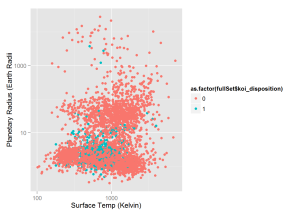This graph plots all KOIs’ planetary radius and the radius of the star the KOI is orbiting. Solar radius is in units of sun radii

``````qplot(x = fullSet\$koi_srad, y = fullSet\$koi_prad,
colour = as.factor(fullSet\$koi_disposition) ,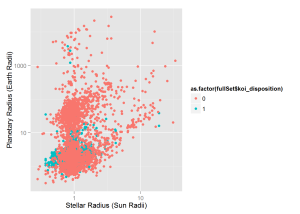The next graph contains only "Confirmed" and "False Positive" KOIs in the goldilocks zone.

``````qplot(x = gold\$koi_teq, y = gold\$koi_prad,
colour = as.factor(gold\$koi_disposition) ,
xlab = 'Surface Temp', ylab = 'Planetary Radius')``````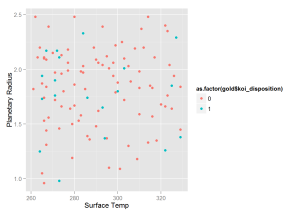The following graph shows all “Candidate” KOIs.

``````qplot(x = newSet\$koi_teq, y = newSet\$koi_prad,
log = 'xy',
xlab = 'Surface Temp', ylab = 'Planetary Radius')``````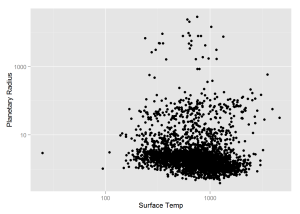And the last graph before I do some predicting is of all "Candidate" KOIs in the goldilocks zone.

``````qplot(x = cangold\$koi_teq, y = cangold\$koi_prad,
xlab = 'Surface Temp', ylab = 'Planetary Radius')``````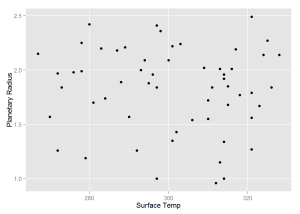To predict the if a KOI is indeed a planet, I will use the random forest model with a threshold of .9 for a positive outcome. First, I predict with the full list of "Candidate" KOIs. The result are then graphed with the color of the data points indicating outcome value. Then I graph only the prediction in the "Goldilocks" Zone.

``````preda = predict(fit.rf,
newdata=newSetCS,
type='response')
pred1 = ifelse(preda > 0.9, 1, 0)
table(newSet\$koi_disposition, pred1)``````
``````##            pred1
##                0    1
##   CANDIDATE 2711  295``````
``````newSet\$koi_disposition = pred1

cangold = newSet[newSet\$koi_teq < 330,]
cangold = cangold[cangold\$koi_teq > 260,]

qplot(x = newSet\$koi_teq, y = newSet\$koi_prad,
colour = as.factor(newSet\$koi_disposition) , log = 'xy',
xlab = 'Surface Temp', ylab = 'Planetary Radius')``````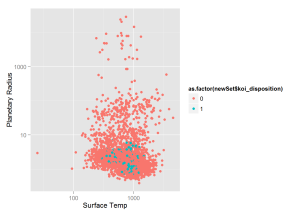``````qplot(x = cangold\$koi_teq, y = cangold\$koi_prad,
colour = as.factor(cangold\$koi_disposition),
xlab = 'Surface Temp', ylab = 'Planetary Radius')``````We see that our model predicts a planet in the "Goldilocks" Zone with probability greater than 0.9. This new planet is potentially habitable for humans beings as it is only roughly twice the size of earth and has an average surface temperature of slightly above freezing. This should be the first planet that NASA attempts to confirm from the "Candidate" list.

### Korrigan Clark

View all articles

Topics from this blog: Student Works

## Interested in becoming a Data Scientist?

Get Customized Course Recommendations In Under a Minute